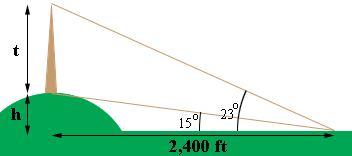SEARCH HOMEMath Central Quandaries & QueriesQuestion from trevor, a student: A tower is on the top of a hill. It is 2,400 feet from an observer to a point directly under the tower and at the same elevation as the observer. The angle of elevation from the observer to the base of the tower is 15 degrees and to the top of the tower 23 degrees. How tall is the tower in feet? Compute your answer within one foot.Hi Trevor,

Here is my diagramThe hill is $h$ feet high and the tower is $t$ fet high.

From the diagram the tangent of 15 degrees is $\frac{h}{2,400}.$ Solve for $h.$

What is the tangent of 23 degrees?

PennyMath Central is supported by the University of Regina and The Pacific Institute for the Mathematical Sciences.